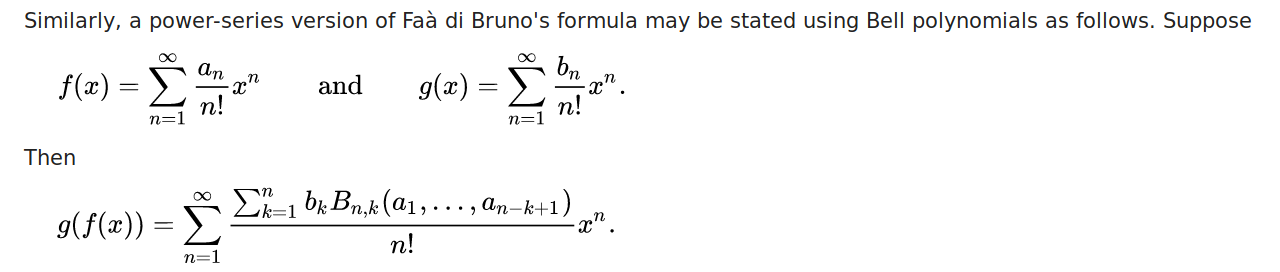Week 2 of the `Coding Period (Phase 1)` is coming to an end. This week was mostly devoted towards polishing the existing `PRs`, and bringing them to a mergeable condition. And this week resulted in the merge of 2 `PRs`. Yayy !!! Here are the deliverables which have been completed in this week. We talked about the remaining work needed to be done in Week 1, and the initial work of Week 2.

• Now, PR #16869, had many loopholes which had to be corrected, before it possibly could have been merged. One issue was that it currently was not able to handle multiple symbols, like `fps of x**(m+n)*sin(x**2)`. So I had to list out all the free symbols in the formal power series, other than `x`, and those, obviously were the symbols used in the expression, which needed to be seperated.

So, I implemented this piece of code –

``````    syms = f.free_symbols.difference({x})
(f, symb) = expand(f).as_independent(*syms)
if symb is S.Zero:
symb = S.One
symb = powsimp(symb)
``````

, which helped us to remove all the symbolic terms, and pass only the non-symbolix term into the `fps` algorithm.

• One more feature implemented in the above PR, were the simplification of terms using `powsimp`. The exponents of the terms are now grouped and printed. Same exists for `ak`, `xk`, and `ind` of the `fps`. Therefore, previously, we had :-
``````  f = x**n*sin(x**2)
assert fps(f, x).truncate(8) == x**2*x**n - x**6*x**n/6 + O(x**(n + 8), x)
``````

But now, we have :-

``````  f = x**n*sin(x**2)
assert fps(f, x).truncate(8) == x**(n + 2) - x**(n + 6)/6 + O(x**(n + 8), x)
``````

After some polishing of the code, like re-using free symbols property of `SeriesBase`, we brought the PR to a mergeable state. Sartaj had a lot of suggestions, and those were duly resolved. The `PR` was ready for review, and it was recently merged (yesterday) !!

• Previously, in `Week 1`, I was looking into Issue 12310, and had come up with a solution. Aaron had already stated in the PR, that we need to create a `Coeff` class to properly indicate the `coefficient sequence of polynomials`. I followed his comments, and published PR #16943. Formerly, polynomial functions, when referenced through `fps`, did not return a `Formal Power Series` object, instead the function itself got returned.
``````>>> from sympy import Symbol, fps
>>> x = Symbol('x')
>>> p = fps(x ** 2)
>>> p
x**2
>>> type(p)
<class 'sympy.core.power.Pow'>
>>> p
Traceback (most recent call last):
File "<stdin>", line 1, in <module>
TypeError: 'Pow' object does not support indexing
``````

I implemented the `Coeff` class according to the lines of Aaron’s comment here. Further integration of class methods and functions might be required into the class. Coefficient sequence of the formal power series of polynomial functions contains a `Coeff` object now.

Now, A `Formal Power Series` object gets returned.

``````>>> from sympy import Symbol, fps
>>> x = Symbol('x')
>>> p = fps(x ** 2)
>>> p
FormalPowerSeries(x**2 + x + 1, x, 0, 1, (SeqFormula(Coeff(x**2 + x + 1, x, _k), (_k, 1, oo)), SeqFormula(x**_k, (_k, 0, oo)), 1))
>>> p.truncate()
1 + x + x**2 + O(x**6)
>>> p
1
``````

This `PR` successfully got approved and merged by Sartaj on 4th June !!

• I also started thinking about the implementation of `convolution`, `composition` and `inversion` of formal power series. A `FormalPowerSeries` function implementation of `def convolve(self, other, x=None, order=4)` is almost completed, and I will publish a `PR` by the next week. It will not return any object, but just the truncated terms upto the specified `order` of the convoluted `fps`. For the `composition` part, I raised a discussion Issue #16975, where I and Sartaj discussed about the way we can implement the composition and inversion. For `composition`, there exists a closed form expression for the resultant power series, where the resultant coefficient sequence `ak` involves Bell polynomials, as follows –Sartaj then provided his perspective in the above issue, and we will discuss about the implementation more about `FiniteFormalPowerSeries` in the upcoming week.

So that was it from Week 2. See you all in the next week !! Adios till then !!